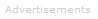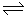# Definition of Equilibrium Constant

What is an Equilibrium Constant?

A reaction's equilibrium constant, Keq, measures the extent to which reactants are converted to products.

Consider the simple reaction equilibrium between A and B: (The symbol ⇌ tells us we are dealing with an equilibrium.)

A ⇌ BEquilibrium does not necessarily mean that the substances are present in equal amounts. It means that the reaction has reached a point where the concentrations of the reactant and product are unchanging with time, because the forward and backward reactions have the same rate.

For the reaction, as it is written above, the equilibrium constant, Keq, is equal to:

Keq = [B]/[A]

The square [ ] brackets indicate the concentration of the chemical species. For example, [A] is the concentration of substance A.

We can deduce the following:

• the higher the equilibrium constant is above 1, the greater the concentration of B relative to A. Reactions that go almost to completion have high equilibrium constants.
• the smaller the equilibrium constant is, as a fraction, between 0 and 1, the greater the concentration of A relative to B. Reactions that barely happen have equilibrium constants that are very small fractional numbers.
• an equilibrium constant equal to 1 means A and B are in equal concentrations.

Useful rules of thumb are:

• If Keq is greater than 105 (i.e. 100,000) the reaction goes to completion.
• If Keq is less than than 10-5 (i.e. 0.00001) the reaction hardly goes at all.A More Formal Definition

Chemical reactions often do not convert all reactants to products. In practice, many reactions reach a state of balance or dynamic equilibrium in which both reactants and products are present.

Equilibrium constants, Keq, are found by multiplying the product concentrations raised to the power of their stoichiometric coefficients divided by the concentrations of reactants raised to the power of their stoichiometric coefficients. This may sound a little complicated, but the examples below show how it works.

Keq measures the extent to which reactants are converted to products in a reaction. Keq is a number that, under specified conditions, is always the same for a given reaction. If the temperature changes, the equilibrium constant will change. This means that when you quote an equilibrium constant, you must also quote the temperature it was obtained at.

## Example of an equilibrium constant for a reaction where there are two reactants and two products

For the balanced reaction:

aA + bBcC + dD

the equilibrium constant is:

```
[C]c [D]d
Keq = -------------
[A]a [B]b ```

Again, the square [ ] brackets indicate the concentration of the chemical species.

## Rules for Writing Keq Expressions

• Products are always in the numerator.

• Reactants are always in the denominator.

• Express gas concentrations as partial pressure, P, and dissolved species in molar concentration, [ ].

• The partial pressures or concentrations are raised to the power of the stoichiometric coefficient for the balanced reaction.

• Ignore pure solids or liquids and any solvent. Only variables will be in a K expression.

### Example of an equilibrium constant for a reaction involving gases and solutes in solution

Zn(s) + 2H+(aq)Zn2+(aq) + H2 (g)

```
[Zn2+] . PH2
K  =  ----------------
[H+]2
```

P is the partial pressure of H2.

### Do equilibrium constants have units?

The correct answer is usually they do. The units are decided by the calculation for Keq. Every time you calculate an equilibrium constant, you need to calculate the units for the equilibrium constant too.

For example, let's look at the gas phase reaction that produces ammonia from hydrogen and nitrogen. The reaction equilibrium is:

N2 (gas) + 3H2 (gas)2NH3 (gas)

The equilibrium constant is:

```
(PNH3)2
Keq =  ----------------
PN2 (PH2)3
```

If the partial pressures of each gas are measured in atmospheres, the units of the equilibrium constant for this reaction will be:

```
atm2
Units =  ----------------
atm . atm3
```

Which simplifies by algebraic cancellation to atm -2

Sometimes the algebraic cancellation will result in the equilibrium constant having no units.

### Equilibrium constants in specific types of reaction

In many instances, rather than seeing the equilibrium constant written in its general way Keq, you will see it written otherwise - in a specific way. For example in gas phase reactions where partial pressures rather than concentrations are used, the equilibrium constant will be written as Kp.

Here are more examples of equilibrium constants with specific names for different classes of reactions:

• Gas-phase reactions that use units of partial pressure: Kp

Dissociation of water: dissociation constant of water, Kw

• Dissociation of acids: acid dissociation constant, Ka

• Reaction of bases with water: base hydrolysis constant, Kb

• Solubility of precipitates: solubility product, Ksp

• Formation of complexes: formation constant, KfSearch the Dictionary for More Terms